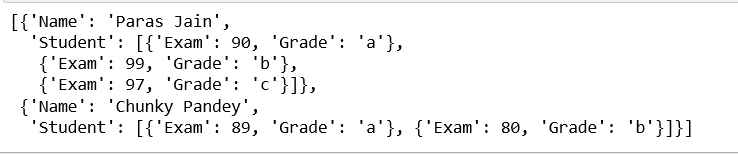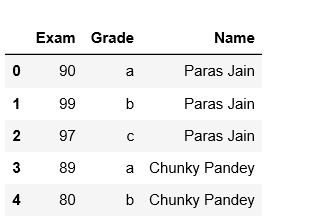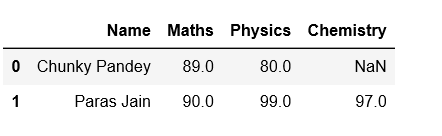# Python | Convert list of nested dictionary into Pandas dataframe

Given a list of nested dictionary, write a Python program to create a Pandas dataframe using it. Let’s understand stepwise procedure to create Pandas Dataframe using list of nested dictionary.

Step #1: Creating a list of nested dictionary.

 `# importing pandas ` `import` `pandas as pd ` ` `  `# List of nested dictionary initialization ` `list` `=` `[ ` `        ``{ ` `        ``"Student"``: [{``"Exam"``: ``90``, ``"Grade"``: ``"a"``}, ` `                    ``{``"Exam"``: ``99``, ``"Grade"``: ``"b"``}, ` `                    ``{``"Exam"``: ``97``, ``"Grade"``: ``"c"``}, ` `                   ``], ` `        ``"Name"``: ``"Paras Jain"` `        ``}, ` `        ``{ ` `        ``"Student"``: [{``"Exam"``: ``89``, ``"Grade"``: ``"a"``}, ` `                    ``{``"Exam"``: ``80``, ``"Grade"``: ``"b"``} ` `                   ``], ` `        ``"Name"``: ``"Chunky Pandey"` `        ``} ` `       ``] ` ` `  `#print(list) `

Output:Step #2: Adding dict values to rows.

 `# rows list initialization ` `rows ``=` `[] ` ` `  `# appending rows ` `for` `data ``in` `list``: ` `    ``data_row ``=` `data[``'Student'``] ` `    ``time ``=` `data[``'Name'``] ` `     `  `    ``for` `row ``in` `data_row: ` `        ``row[``'Name'``]``=` `time ` `        ``rows.append(row) ` ` `  `# using data frame ` `df ``=` `pd.DataFrame(rows) ` ` `  `# print(df) `

Output:Step #3: Pivoting dataframe and assigning column names.

 `# using pivot_table ` `df ``=` `df.pivot_table(index ``=``'Name'``, columns ``=``[``'Grade'``], ` `                        ``values ``=``[``'Exam'``]).reset_index() ` ` `  `# Defining columns ` `df.columns ``=``[``'Name'``, ``'Maths'``, ``'Physics'``, ``'Chemistry'``] ` ` `  `# print dataframe ` `print``(df) `

Output:Below is the complete code:

 `# Python program to convert list of nested  ` `# dictionary into Pandas dataframe ` ` `  `# importing pandas ` `import` `pandas as pd ` ` `  `# List of list of dictionary initialization ` `list` `=` `[ ` `        ``{ ` `        ``"Student"``: [{``"Exam"``: ``90``, ``"Grade"``: ``"a"``}, ` `                    ``{``"Exam"``: ``99``, ``"Grade"``: ``"b"``}, ` `                    ``{``"Exam"``: ``97``, ``"Grade"``: ``"c"``}, ` `                   ``], ` `        ``"Name"``: ``"Paras Jain"` `        ``}, ` `        ``{ ` `        ``"Student"``: [{``"Exam"``: ``89``, ``"Grade"``: ``"a"``}, ` `                    ``{``"Exam"``: ``80``, ``"Grade"``: ``"b"``} ` `                  ``], ` `        ``"Name"``: ``"Chunky Pandey"` `        ``} ` `       ``] ` ` `  `# rows list initialization ` `rows ``=` `[] ` ` `  `# appending rows ` `for` `data ``in` `list``: ` `    ``data_row ``=` `data[``'Student'``] ` `    ``time ``=` `data[``'Name'``] ` `     `  `    ``for` `row ``in` `data_row: ` `        ``row[``'Name'``]``=` `time ` `        ``rows.append(row) ` ` `  `# using data frame ` `df ``=` `pd.DataFrame(rows) ` ` `  `# using pivot_table ` `df ``=` `df.pivot_table(index ``=``'Name'``, columns ``=``[``'Grade'``], ` `                        ``values ``=``[``'Exam'``]).reset_index() ` ` `  `# Defining columns ` `df.columns ``=``[``'Name'``, ``'Maths'``, ``'Physics'``, ``'Chemistry'``] ` ` `  `# print dataframe ` `print``(df) `

Output:

```            Name  Maths  Physics  Chemistry
0  Chunky Pandey     89       80        NaN
1     Paras Jain     90       99         97```

My Personal Notes arrow_drop_upIf you like GeeksforGeeks and would like to contribute, you can also write an article using contribute.geeksforgeeks.org or mail your article to contribute@geeksforgeeks.org. See your article appearing on the GeeksforGeeks main page and help other Geeks.

Please Improve this article if you find anything incorrect by clicking on the "Improve Article" button below.

Improved By : shubham_singh

Article Tags :

Be the First to upvote.

Please write to us at contribute@geeksforgeeks.org to report any issue with the above content.